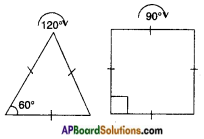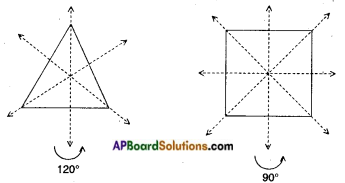# AP Board 7th Class Maths Notes Chapter 15 Symmetry

Students can go through AP Board 7th Class Maths Notes Chapter 15 Symmetry to understand and remember the concepts easily.

## AP State Board Syllabus 7th Class Maths Notes Chapter 15 Symmetry

→ Line of symmetry: The line which divides a figure into two identical parts is called the line of symmetry or axis of symmetry.
Ex: In the adjacent figure the dotted lines are the line of symmetry.→ An object can have one or more than one lines of symmetry or axes of symmetry.
Ex: In the above figure there are two lines of symmetry.→ If we rotate a figure, about a fixed point by a certain angle and the figure looks exactly the same as before, we say that the figure has rotational symmetry.
Ex: An equilateral triangle; a square etc.→ The angle of turning during rotation is called the angle of rotation (or) the minimum angle rotation of a figure to get exactly the same figure as original is called the angle of rotation.
Ex: i) Angle of rotation of an equilateral triangle = 120°.
ii) Angle of rotation of a square = 90°.

→ All figures having rotational symmetry of order 1, can be rotated completely through 360° to come back to their original position. So we say that an object has rotational symmetry only when the order of symmetry is more than 1.
Eg: The order of rotational symmetry for an equilateral triangle is 3.
ii) For a square is 4.→ Some shapes only have line symmetry and some have only rotational symmetry and some have both. Squares, equilateral triangles and circles have both line symmetry and rotational symmetry.